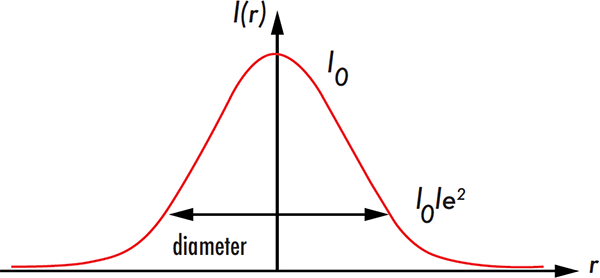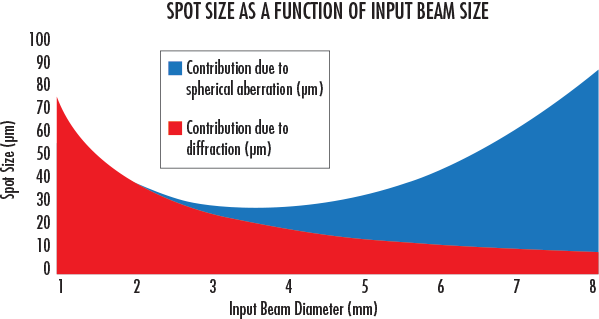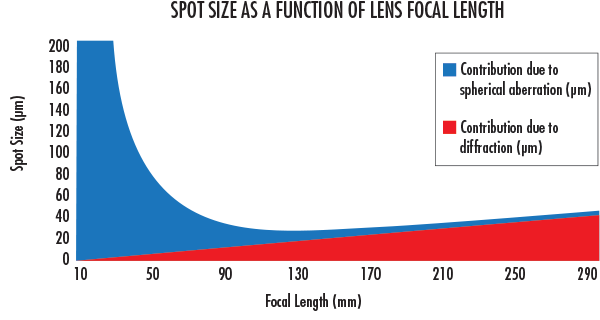# Advantages of Using Beam Expanders

Focusing a laser beam down to a small spot is an integral part of many laser optical applications. While some applications benefit from large spot sizes, most require the smallest spot possible.

The spot size is usually defined as the radial distance from the center point of maximum irradiance to point where the intensity drops to 1/e2 of the initial value.

(1)$$\definecolor{Diffraction}{RGB}{0, 0, 255} \definecolor{Aberration}{RGB}{255, 0, 0} \phi_{\text{Spot Size}} = \color{Diffraction} \phi_{\text{Diffraction}} \color{black} + \color{Aberration} \phi_{\text{Aberration}} \color{black} = \color{Diffraction} \frac{4 \lambda M^2 f}{\pi D} \color{black} + \color{Aberration} \frac{k D^3}{f^2}$$

λ:  Represents the wavelength
f:  Represents the focal length of your lens
D:  Represents the diameter of the input beam
k:  Represents the index of refraction of your lens
M2:  Represents the beam quality factor, this represents the degree of variation from an ideal Gaussian beam##### Figure 1: The spot size is usually defined as the radial distance from the center point of maximum irradiance to point where the intensity drops to 1/e2 of the initial value.

Spot size is fundamentally determined by the combination of diffraction (red) and aberrations (blue). In this example we can assume that spherical aberration is the dominant aberration, and consider it the only type of aberration. For diffraction, we see that the shorter the focal length, the smaller the spot size. More importantly, the larger the input beam diameter, the smaller the spot size. This shows the advantage of using beam expanders in laser optical applications.

By expanding the beam within the system, the input beam diameter D is increased by a factor m, reducing the divergence by a factor m. When the beam is finally focused down to a small spot, this spot is a factor of m smaller than for the unexpanded beam for an ideal, diffraction limited spot. There is however a tradeoff with spherical aberration, which increases as the input beam diameter increases.##### Figure 2: At small input beam diameters, the spot size is diffraction limited. As the input beam diameter increases, spherical aberration starts to dominate the spot size. This plot was generated using a wavelength of 532nm, M2 factor 1.1, of focal length of 10mm, and refractive index of 1.3.